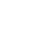Live chatQuestion 1: Compute the revenue, variable expense and contribution margin for each cruise.

Revenue value:

The revenue is determined by multiplying the quantity by the price, in this case the quantity value is equal to the number of tourist. Therefore Revenue = P*Q.

When calculating the revenue where P = \$100 and Q = 30

Revenue = \$100*30 = \$3,000

Revenue =\$3,000

## Buy Free Custom «Contribution Margin and Revenue» Essay Paper paper online

* Final order price might be slightly different depending on the current exchange rate of chosen payment system.

Variable expenses value:

The variable expenses include:

Labor expenses- include 6 experienced university graduates:

For each trip 6 are required

Each is paid \$100 per trip

Therefore for each trip the total labor cost is:

Labor cost = number of employed*cost per unit

Labor cost = 6*\$100

Labor cost = \$600

Refreshments:

Tourist are offered refreshments, the cost per person is \$25

Refreshments cost = \$25

Total refreshments cost = cost per unit * number of tourist

Number of tourists = 30

Total refreshment cost = \$25*30

Stay ConnectedTotal refreshment cost = \$450

Other operating expenses (including fuel cost)

Other expenses =\$50 per trip

Total expenses = labor expenses + refreshment costs + other operating expenses

Total expenses = (6*\$100) + (\$25*30) + (\$50)

Total expenses = \$600+\$450+\$50

Total expenses =\$1,100

Contribution margin:

The difference between total revenue and total variable expenses is referred to as the contribution margin, in this case given the total number of tourists = 30

Total revenue = \$3000

Total variable expenses = \$1,100

Contribution margin = 3,000 - 1,100

Contribution margin for each trip is = \$1,900

Limited time Offer

0
0
days
:
0
0
hours
:
0
0
minutes
:
0
0
seconds
Get 19% OFF

From the above calculations the following values were determined:

Total revenue = \$3,000

Total variable expenses = \$1,100

Contribution margin = \$1,900

This means that given the average number of tourists per cruise was 30, the revenue per cruise was \$3,000. Also given the same number of tourists the variable expenses per cruise was \$1,100 and given these two values the contribution margin was \$1,900 per cruise.

0

Preparing Orders

0

Active Writers

0

Support Agents

Limited offerget 15% off your 1st order